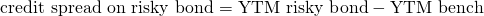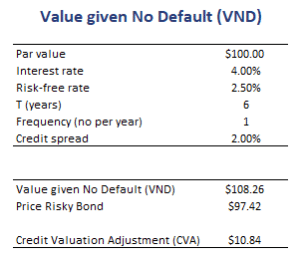# Value Given No Default (VND)

The Value given No Default (VND) is the theoretical price of a bond assuming the bond is not exposed to default risk. Thus, it is the price of the bond if it were default-free. The VND is a very useful concept that can easily be calculated. We only need two ingredients. First, calculating the VND requires the appropriate set of risk-free rates. Second, we should know how to discount cash flows. The VND is very useful when it comes to calculating the credit valuation adjustment (CVA).

On this page, we show you how to calculate the value given no default using spot rate curve, how to use it to determine the CVA, and finally conclude with an example. The Excel spreadsheet that implements the example is available at the bottom of the page.

## Value given No Default definition

The VND is calculated using the risk-free rate to value the bond. Thus, we should discount the coupon payments and the principal at maturity at the risk-free rate. Of course, the actual yield to maturity (YTM) of the bond does not equal the risk-free rate. Instead, they differ by the credit spreadNote that if we want to introduce volatility to this framework, we should use the backward induction process to value the risky bond given the benchmark risk-free rate tree.

## Value given No Default example

Next, let’s discuss a VND example. In particular, let’s assume we have a bond with the properties as displayed in the table and a yield curve that is flat. In that case, we can calculate the VND as followsThe figure illustrates that calculating the VND is nothing more than discounting the risky bond at the risk-free rate. Using the actual price of the risky bond in the market, we can then calculate the credit valuation adjustment (CVA).

## Summary

We discussed the VND. It can be used to calculate the credit valuation adjustment. That is, the difference between the price on a risky bond and the price on an otherwise identical risk-free bond.